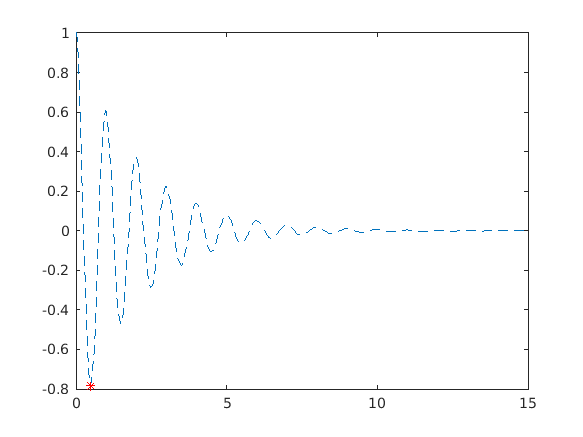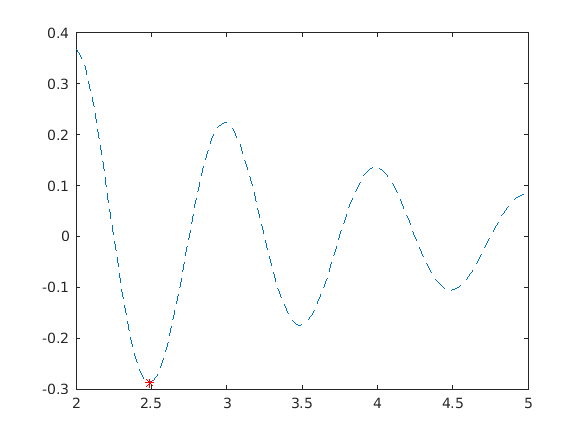Cody

# Problem 44934. Plot Damped Sinusoid

Solution 2059232

Submitted on 16 Dec 2019
This solution is locked. To view this solution, you need to provide a solution of the same size or smaller.

### Test Suite

Test Status Code Input and Output
1   Fail
clf; t = linspace(0,15,400); y = exp(-0.5*t).*cos(2*pi.*t); m = plot_cos(y, t); assert(abs(m - (-0.781239288889930)) <= 1e-4) h = findobj(gcf, 'Type', 'Line'); if length(h) == 2 assert(isequal([h.Color], [1 0 0 0 0 1]), 'Check plot colors') assert(strcmp([h.LineStyle], 'none--'), 'Check the line style') assert(strcmp([h.Marker],'*none'), 'Check marker type') assert(isequal([h.YData],[m, y]), 'Check plotted data') elseif length(h) == 1 assert(isequal([h.Color], [0 0 1]),'Check plot colors') assert(strcmp([h.LineStyle], '--'), 'Check the line style') assert(strcmp([h.Marker],'*'),'Check marker type') assert(isequal([h.YData],y),'Check plotted data' ) assert(isequal([h.MarkerEdgeColor], [1 0 0]),'Check plot colors') assert(isequal([h.MarkerIndices], 14),'Check marker location') elseif length(h)>2 error('There may be too many plots on the figure.') else error('No plot found.') end

Undefined function 'minus' for input arguments of type 'matlab.graphics.chart.primitive.Line'. Error in Test1 (line 5) assert(abs(m - (-0.781239288889930)) <= 1e-4)

``
2   Fail
clf; t = linspace(2,5,100); y = exp(-0.5*t).*cos(2*pi.*t); m = plot_cos(y, t); assert(abs(m - (-0.287376348726584)) <= 1e-4) h = findobj(gcf, 'Type', 'Line'); if length(h) == 2 assert(isequal([h.Color], [1 0 0 0 0 1]), 'Check plot colors') assert(strcmp([h.LineStyle], 'none--'), 'Check the line style') assert(strcmp([h.Marker],'*none'), 'Check marker type') assert(isequal([h.YData],[m, y]), 'Check plotted data') elseif length(h) == 1 assert(isequal([h.Color], [0 0 1]),'Check plot colors') assert(strcmp([h.LineStyle], '--'), 'Check the line style') assert(strcmp([h.Marker],'*'),'Check marker type') assert(isequal([h.YData],y),'Check plotted data' ) assert(isequal([h.MarkerEdgeColor], [1 0 0]),'Check plot colors') assert(isequal([h.MarkerIndices],17 ),'Check marker location') elseif length(h)>2 error('There may be too many plots on the figure.') else error('No plot found.') end

Undefined function 'minus' for input arguments of type 'matlab.graphics.chart.primitive.Line'. Error in Test2 (line 5) assert(abs(m - (-0.287376348726584)) <= 1e-4)

``

### Community Treasure Hunt

Find the treasures in MATLAB Central and discover how the community can help you!

Start Hunting!